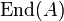# Endomorphism ring of an abelian group

(diff) ← Older revision | Latest revision (diff) | Newer revision → (diff)
Jump to: navigation, search

## Definition

Suppose$A$ is an abelian group. The endomorphism ring of$A$, denoted$\operatorname{End}(A)$ is defined as follows:

1. As a set, it is the set of all endomorphisms of$A$.
2. The addition is pointwise addition in the target group. In other words, for endomorphisms$f,g$ of$A$, we define$f + g$ as the map$a \mapsto f(a) + g(a)$. Thus, the additive identity is the zero map (the map sending everything to zero) and the negation is the pointwise negation.
3. The multiplication is given by function composition. In other words,$fg$ is the map sending$a$ to$f(g(a))$. The identity for multiplication is the identity map.

## Facts

• For a non-abelian group, the pointwise group multiplication of two endomorphisms need not be an endomorphism.
• For functions that are not endomorphisms, only one of the distributivity laws holds in general.